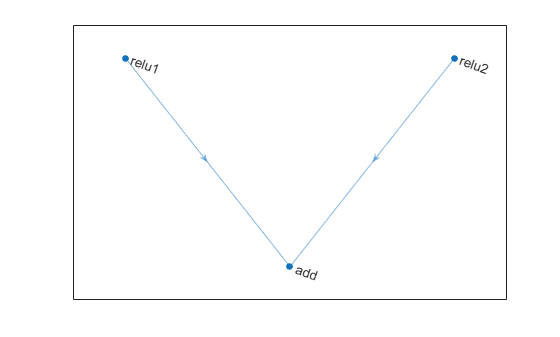## Description

Specify the number of inputs to the layer when you create it. The inputs to the layer have the names `'in1','in2',...,'inN'`, where `N` is the number of inputs. Use the input names when connecting or disconnecting the layer by using `connectLayers` or `disconnectLayers`. All inputs to an addition layer must have the same dimension.

## Creation

### Syntax

``layer = additionLayer(numInputs)``
``layer = additionLayer(numInputs,'Name',name)``

### Description

example

````layer = additionLayer(numInputs)` creates an addition layer that adds `numInputs` inputs element-wise. This function also sets the `NumInputs` property.```

example

````layer = additionLayer(numInputs,'Name',name)` also sets the `Name` property.```

## Properties

expand all

Number of inputs to the layer, specified as a positive integer greater than or equal to 2.

The inputs have the names `'in1','in2',...,'inN'`, where `N` is `NumInputs`. For example, if `NumInputs` is 3, then the inputs have the names `'in1','in2'`, and `'in3'`. Use the input names when connecting or disconnecting the layer using the `connectLayers` or `disconnectLayers` functions.

Layer name, specified as a character vector or a string scalar. For `Layer` array input, the `trainnet`, `trainNetwork`, `assembleNetwork`, `layerGraph`, and `dlnetwork` functions automatically assign names to layers with the name `""`.

The `AdditionLayer` object stores this property as a character vector.

Data Types: `char` | `string`

Input names, specified as `{'in1','in2',...,'inN'}`, where `N` is the number of inputs of the layer.

Data Types: `cell`

Number of outputs from the layer, returned as `1`. This layer has a single output only.

Data Types: `double`

Output names, returned as `{'out'}`. This layer has a single output only.

Data Types: `cell`

## Examples

collapse all

Create an addition layer with two inputs and the name `'add_1'`.

`add = additionLayer(2,'Name','add_1')`
```add = AdditionLayer with properties: Name: 'add_1' NumInputs: 2 InputNames: {'in1' 'in2'} ```

Create two ReLU layers and connect them to the addition layer. The addition layer sums the outputs from the ReLU layers.

```relu_1 = reluLayer('Name','relu_1'); relu_2 = reluLayer('Name','relu_2'); lgraph = layerGraph; lgraph = addLayers(lgraph,relu_1); lgraph = addLayers(lgraph,relu_2); lgraph = addLayers(lgraph,add); lgraph = connectLayers(lgraph,'relu_1','add_1/in1'); lgraph = connectLayers(lgraph,'relu_2','add_1/in2'); plot(lgraph)```Create a simple directed acyclic graph (DAG) network for deep learning. Train the network to classify images of digits. The simple network in this example consists of:

• A main branch with layers connected sequentially.

• A shortcut connection containing a single 1-by-1 convolutional layer. Shortcut connections enable the parameter gradients to flow more easily from the output layer to the earlier layers of the network.

Create the main branch of the network as a layer array. The addition layer sums multiple inputs element-wise. Specify the number of inputs for the addition layer to sum. To easily add connections later, specify names for the first ReLU layer and the addition layer.

```layers = [ imageInputLayer([28 28 1]) convolution2dLayer(5,16,'Padding','same') batchNormalizationLayer reluLayer('Name','relu_1') convolution2dLayer(3,32,'Padding','same','Stride',2) batchNormalizationLayer reluLayer convolution2dLayer(3,32,'Padding','same') batchNormalizationLayer reluLayer additionLayer(2,'Name','add') averagePooling2dLayer(2,'Stride',2) fullyConnectedLayer(10) softmaxLayer classificationLayer];```

Create a layer graph from the layer array. `layerGraph` connects all the layers in `layers` sequentially. Plot the layer graph.

```lgraph = layerGraph(layers); figure plot(lgraph)```Create the 1-by-1 convolutional layer and add it to the layer graph. Specify the number of convolutional filters and the stride so that the activation size matches the activation size of the third ReLU layer. This arrangement enables the addition layer to add the outputs of the third ReLU layer and the 1-by-1 convolutional layer. To check that the layer is in the graph, plot the layer graph.

```skipConv = convolution2dLayer(1,32,'Stride',2,'Name','skipConv'); lgraph = addLayers(lgraph,skipConv); figure plot(lgraph)```Create the shortcut connection from the `'relu_1'` layer to the `'add'` layer. Because you specified two as the number of inputs to the addition layer when you created it, the layer has two inputs named `'in1'` and `'in2'`. The third ReLU layer is already connected to the `'in1'` input. Connect the `'relu_1'` layer to the `'skipConv'` layer and the `'skipConv'` layer to the `'in2'` input of the `'add'` layer. The addition layer now sums the outputs of the third ReLU layer and the `'skipConv'` layer. To check that the layers are connected correctly, plot the layer graph.

```lgraph = connectLayers(lgraph,'relu_1','skipConv'); lgraph = connectLayers(lgraph,'skipConv','add/in2'); figure plot(lgraph);```Load the training and validation data, which consists of 28-by-28 grayscale images of digits.

```[XTrain,YTrain] = digitTrain4DArrayData; [XValidation,YValidation] = digitTest4DArrayData;```

Specify training options and train the network. `trainNetwork` validates the network using the validation data every `ValidationFrequency` iterations.

```options = trainingOptions('sgdm', ... 'MaxEpochs',8, ... 'Shuffle','every-epoch', ... 'ValidationData',{XValidation,YValidation}, ... 'ValidationFrequency',30, ... 'Verbose',false, ... 'Plots','training-progress'); net = trainNetwork(XTrain,YTrain,lgraph,options);```Display the properties of the trained network. The network is a `DAGNetwork` object.

`net`
```net = DAGNetwork with properties: Layers: [16x1 nnet.cnn.layer.Layer] Connections: [16x2 table] InputNames: {'imageinput'} OutputNames: {'classoutput'} ```

Classify the validation images and calculate the accuracy. The network is very accurate.

```YPredicted = classify(net,XValidation); accuracy = mean(YPredicted == YValidation)```
```accuracy = 0.9934 ```

expand all

## Version History

Introduced in R2017b